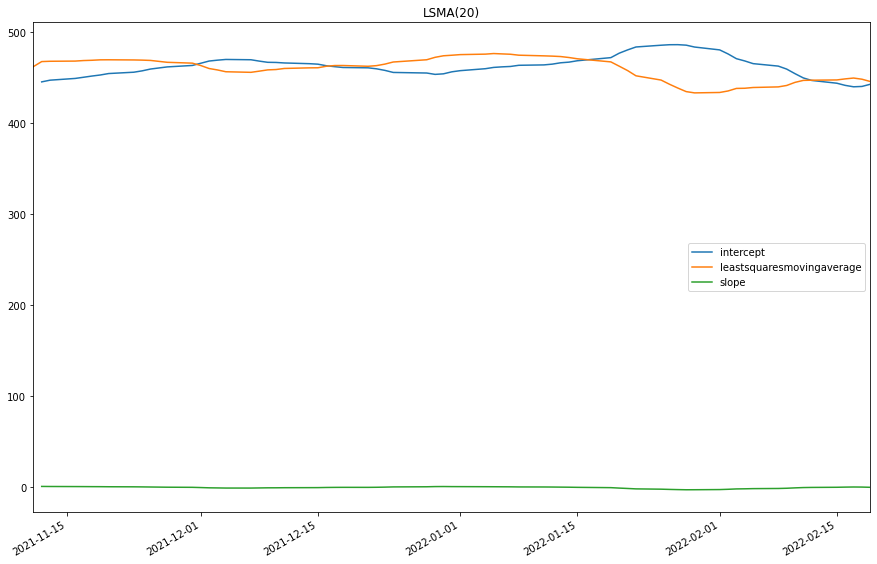# Indicator Reference

## Least Squares Moving Average

### Introduction

The Least Squares Moving Average (LSMA) first calculates a least squares regression line over the preceding time periods, and then projects it forward to the current period. In essence, it calculates what the value would be if the regression line continued. source

### Create Manual Indicators

You can manually create a LeastSquaresMovingAverage indicator, so it doesn’t automatically update. Manual indicators let you update their values with any data you choose. The following reference table describes the LeastSquaresMovingAverage constructor.

### LeastSquaresMovingAverage()1/2

            LeastSquaresMovingAverage QuantConnect.Indicators.LeastSquaresMovingAverage (
string  name,
int     period
)


Initializes a new instance of the LeastSquaresMovingAverage class.

### LeastSquaresMovingAverage()2/2

            LeastSquaresMovingAverage QuantConnect.Indicators.LeastSquaresMovingAverage (
int  period
)


Initializes a new instance of the LeastSquaresMovingAverage class.

### Update Manual Indicators

You can update the indicator automatically or manually.

#### Automatic Update

To register a manual indicator for automatic updates with the security data, call the RegisterIndicator method.


private LeastSquaresMovingAverage _lsma;

// In Initialize()
_lsma = new LeastSquaresMovingAverage(name, period);
_lsma.Updated += IndicatorUpdateMethod;

RegisterIndicator(symbol, _lsma, Resolution.Daily);

// In IndicatorUpdateMethod()
{
var indicatorValue = _lsma.Current.Value;
}

# In Initialize()
self.lsma = LeastSquaresMovingAverage(name, period)
self.lsma.Updated += self.IndicatorUpdateMethod

self.RegisterIndicator(symbol, self.lsma, Resolution.Daily)

# In IndicatorUpdateMethod()
indicator_value = self.lsma.Current.Value

To customize the data that automatically updates the indicator, see Custom Indicator Periods and Custom Indicator Values.

#### Manual Update

Updating your indicator manually enables you to control when the indicator is updated and what data you use to update it. To manually update the indicator, call the Update method with time/decimal pair. The indicator will only be ready after you prime it with enough data.


private LeastSquaresMovingAverage _lsma;
private Symbol symbol;

// In Initialize()
_lsma = new LeastSquaresMovingAverage(period);

// In OnData()
if (data.ContainsKey(_symbol))
{
_lsma.Update(data[symbol].EndTime, data[symbol].High);
}
{
var indicatorValue = _lsma.Current.Value;
}

# In Initialize()
self.lsma = LeastSquaresMovingAverage(period)

# In OnData()
if data.ContainsKey(self.symbol):
self.lsma.Update(data[self.symbol].EndTime, data[self.symbol].High)
indicator_value = self.lsma.Current.Value

### Create Automatic Indicators

The LSMA method creates an LeastSquaresMovingAverage indicator, sets up a consolidator to update the indicator, and then returns the indicator so you can use it in your algorithm.

The following reference table describes the LSMA method:

### LSMA()1/1

            LeastSquaresMovingAverage QuantConnect.Algorithm.QCAlgorithm.LSMA (
Symbol                           symbol,
Int32                            period,
*Nullable<Resolution>      resolution,
*Func<IBaseData, Decimal>  selector
)


Creates and registers a new Least Squares Moving Average instance.

If you don't provide a resolution, it defauls to the security resolution. If you provide a resolution, it must be greater than or equal to the resolution of the security. For instance, if you subscribe to hourly data for a security, you should update its indicator with data that spans 1 hour or longer.

### Get Indicator Values

To get the value of the indicator, use its Current.Value attribute.

private LeastSquaresMovingAverage _lsma;

// In Initialize()
_lsma = LSMA(symbol, period);

// In OnData()
{
var intercept = _lsma.Intercept.Current.Value;
var slope = _lsma.Slope.Current.Value;
var current = _lsma.Current.Value;
}
# In Initialize()
self.lsma = self.LSMA(symbol, period)

# In OnData()
intercept = self.lsma.Intercept.Current.Value
slope = self.lsma.Slope.Current.Value
current = self.lsma.Current.Value


### Visualization

To plot indicator values, in the OnData event handler, call the Plot method.

private LeastSquaresMovingAverage _lsma;

// In Initialize()
_lsma = LSMA(symbol, period);

// In OnData()
{
Plot("My Indicators", "intercept", _lsma.Intercept);
Plot("My Indicators", "slope", _lsma.Slope);
Plot("My Indicators", "leastsquaresmovingaverage", _lsma.Current);
}
# In Initialize()
self.lsma = self.LSMA(symbol, period)

# In OnData()